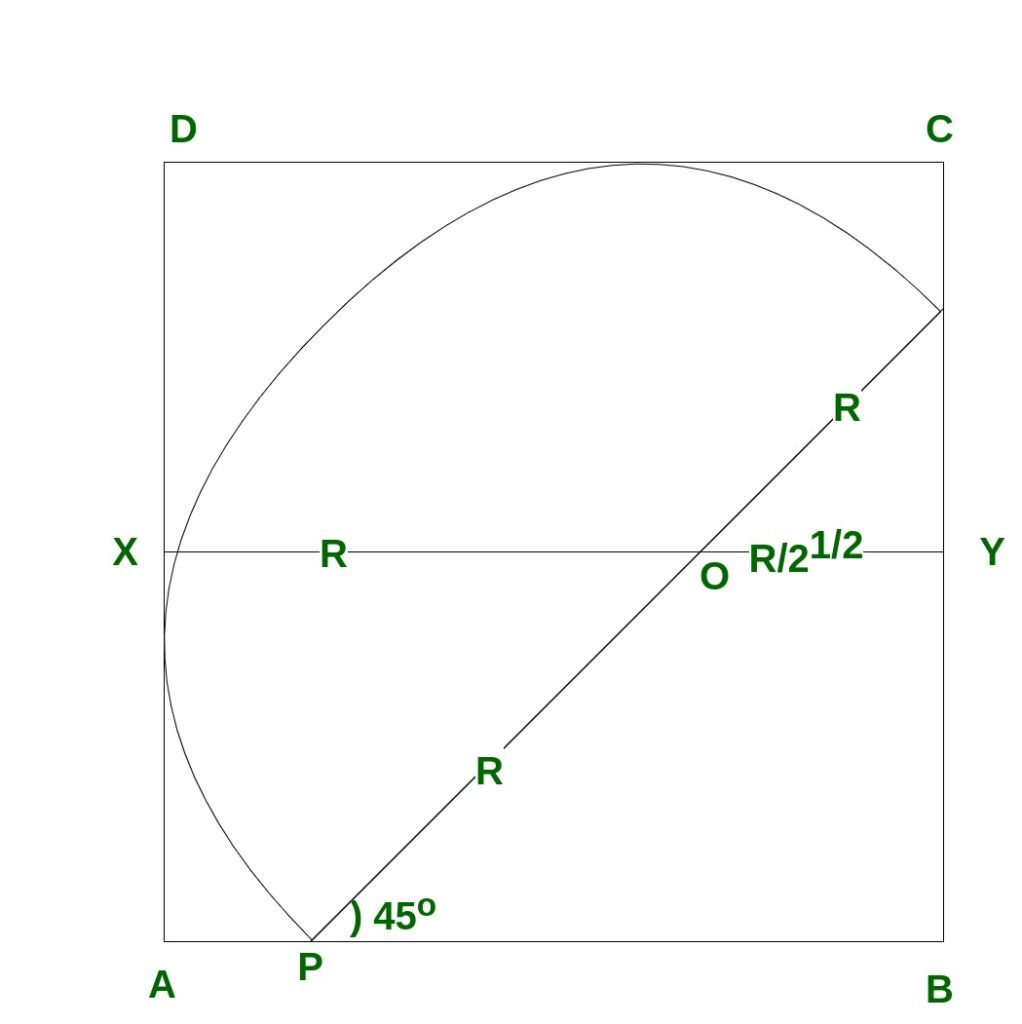# Area of largest semicircle that can be drawn inside a square

Given a square of side a, the task is to find the area of the largest semi-circle that can be drawn inside the square.

Examples:

```Input: a = 3
Output: 4.84865

Input: a = 4
Output: 8.61982
```

## Recommended: Please try your approach on {IDE} first, before moving on to the solution.

Approach
The semicircle of maximal area inscribed in the square has its diameter parallel to a diagonal, and its radius rmax is given as:• Since the figure is symmetrical in the diagonal BD, angle QPB = 45°.
`OY = r cos 45 = r/ √2`
• Hence
```a = AB
= r + r/√2
= r(1 + 1/√2)
```
• Thus
```r = a / (1 + 1/√2)
= a*√2 / (√2 + 1)
```
• Rationalizing the denominator, we obtain
`r = a*√2*(√2-1)`
• Thus
```r = a*2 - a √2
= a*(2-√2)
```
• Therfore,
```Area of the required semicircle
= pi * r2/2
= 3.14*(a*(2-√2))2 / 2
```

Below is the implementation of the above approach:

## CPP

 `// C++ program to find Area of ` `// semicircle in a square ` ` `  `#include ` `using` `namespace` `std; ` ` `  `// Function to find area of semicircle ` `float` `find_Area(``float` `a) ` `{ ` `    ``float` `R = a * (2.0 - ``sqrt``(2)); ` `    ``float` `area = 3.14 * R * R / 2.0; ` `    ``return` `area; ` `} ` ` `  `// Driver code ` `int` `main() ` `{ ` `    ``// side of a square ` `    ``float` `a = 4; ` ` `  `    ``// Call Function to find ` `    ``// the area of semicircle ` `    ``cout << ``" Area of semicircle = "` `         ``<< find_Area(a); ` ` `  `    ``return` `0; ` `} `

## Java

 `// Java program to find Area of ` `// semicircle in a square ` `class` `GFG { ` ` `  `    ``// Function to find area of semicircle ` `    ``static` `float` `find_Area(``float` `a) ` `    ``{ ` `        ``float` `R = a * (``float``)(``2.0` `- Math.sqrt(``2``)); ` `        ``float` `area = (``float``)((``3.14` `* R * R) / ``2.0``); ` `        ``return` `area; ` `    ``} ` `     `  `    ``// Driver code ` `    ``public` `static` `void` `main (String[] args) ` `    ``{ ` `        ``// side of a square ` `        ``float` `a = ``4``; ` `     `  `        ``// Call Function to find ` `        ``// the area of semicircle ` `        ``System.out.println(``" Area of semicircle = "` `+ find_Area(a)); ` `    ``} ` `} ` ` `  `// This code is contributed by AnkitRai01 `

## Python3

 `# Python3 program to find Area of ` `# semicircle in a square ` `from` `math ``import` `sqrt ` ` `  `# Function to find area of semicircle ` `def` `find_Area(a) : ` ` `  `    ``R ``=` `a ``*` `(``2.0` `-` `sqrt(``2``)); ` `    ``area ``=` `3.14` `*` `R ``*` `R ``/` `2.0``; ` `     `  `    ``return` `area; ` ` `  `# Driver code ` `if` `__name__ ``=``=` `"__main__"` `: ` ` `  `    ``# side of a square ` `    ``a ``=` `4``; ` ` `  `    ``# Call Function to find ` `    ``# the area of semicircle ` `    ``print``(``"Area of semicircle ="``,find_Area(a)); ` ` `  `# This code is contributed by AnkitRai01 `

## C#

 `// C# program to find Area of ` `// semicircle in a square ` `using` `System; ` ` `  `class` `GFG { ` ` `  `    ``// Function to find area of semicircle ` `    ``static` `float` `find_Area(``float` `a) ` `    ``{ ` `        ``float` `R = a * (``float``)(2.0 - Math.Sqrt(2)); ` `        ``float` `area = (``float``)((3.14 * R * R) / 2.0); ` `        ``return` `area; ` `    ``} ` `     `  `    ``// Driver code ` `    ``public` `static` `void` `Main (``string``[] args) ` `    ``{ ` `        ``// side of a square ` `        ``float` `a = 4; ` `     `  `        ``// Call Function to find ` `        ``// the area of semicircle ` `        ``Console.WriteLine(``" Area of semicircle = "` `+ find_Area(a)); ` `    ``} ` `} ` ` `  `// This code is contributed by AnkitRai01 `

Output:

```Area of semicircle = 8.61982
```

Reference: http://www.qbyte.org/puzzles/p153s.html

Attention reader! Don’t stop learning now. Get hold of all the important DSA concepts with the DSA Self Paced Course at a student-friendly price and become industry ready.

My Personal Notes arrow_drop_upCheck out this Author's contributed articles.

If you like GeeksforGeeks and would like to contribute, you can also write an article using contribute.geeksforgeeks.org or mail your article to contribute@geeksforgeeks.org. See your article appearing on the GeeksforGeeks main page and help other Geeks.

Please Improve this article if you find anything incorrect by clicking on the "Improve Article" button below.

Improved By : AnkitRai01